# Plotting data

You can plot data using `plot()`. This can be used in several ways. The simplest is to plot some numeric x-values against some numeric y-values using:

`plot( x.variable.vector, y.variable.vector )`

For example:

```x<-c( 1:10 )
y<-1 + 2*x
plot( x, y )```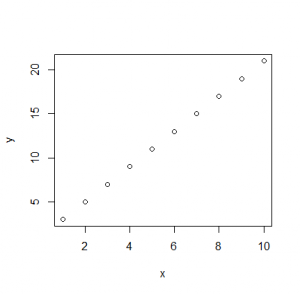However, for data you have imported into a data frame, the x and y variables are now named columns in a data frame, rather than separate vectors. To plot data in data frames, there are two options. The first is to use the dollar \$ syntax to access the vector of data within the data frame (here sycamore_seeds.csv) by its column name:

```sycamore.seeds<-read.csv( "H:/R/sycamore_seeds.csv")

# You'll need to use whatever column names you chose:
names( sycamore.seeds )```
` "wing.length"  "descent.speed"`
`plot( sycamore.seeds\$wing.length, sycamore.seeds\$descent.speed )`The second is to use:

`plot( y.name ~ x.name, data = your.data.frame )`

You can read the tilde ~ as modelled on. We’ll come across many other uses of the modelled-on tilde in later posts.

`plot( descent.speed ~ wing.length, data = sycamore.seeds )`

“Using the data in the `sycamore.seeds` data frame, use the `descent.speed` column as the dependent variable, and model it using the `wing.length` column as the independent variable”

As both variables are numeric, R will assume you want to `plot()` this model as a simple x,y-scatterplot: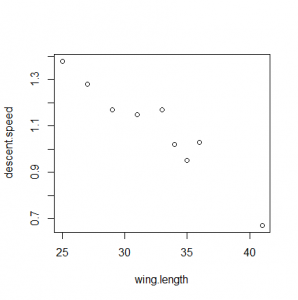Graphs ought to have better axis labels and more descriptive titles than this, which you can also control using `name=value` named argument pairs, namely, `xlab`, `ylab` and `main`:

```plot(
descent.speed ~ wing.length,
data = sycamore.seeds,
xlab = "Wing length / mm",
ylab = expression("Descent speed" / m*s^-1),
main = "Sycamore seeds with longer wings fall more slowly"
)```

These labels can either be `"simple strings"` or they can be an `expression()`. Where possible, it’s easiest to use strings. However, you won’t have any choice but to use `expression()` if you need super/sub scripts or italic text. An `expression()` can include any number of the following components (`demo(plotmath)` for more):

• `"A string"`
• `something^superscripted`
• `something[subscripted]`
• `x-an*algebraic/expression+y`
• `"A string " * " masquerading as a multiplication using a * to juxtapose two items"`
• `"A string containing a micro µ sign: you can use ASCII characters freely"`
• `greek*symbols/pi*get-transcribed*alpha`
• `italic("Latin name for example")`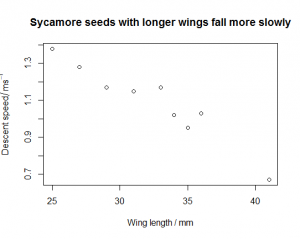Most graphs also support options such as:

• Differently coloured data points: `col="red"`
• Different plot characters: `pch=15` (see `help(points)` for details)

These and many other graphical options are explained in `help(par)`.

For data where the dependent variable is numeric, and the independent variable is categorical, R will plot the data as a box-and-whisker plot rather than an x,y-scatterplot. You can also force R to do this using `boxplot()` with the same parameters.

```dog.whelks<-read.csv("H:/R/dog_whelks.csv")
boxplot(
Height ~ Exposure,
data = dog.whelks,
xlab = "Beach",
ylab = "Shell height / mm",
main = "Dog whelks have taller shells on sheltered beaches"
)```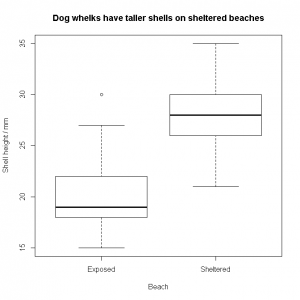In a box-and-whisker plot:

• The black bar represents the median
• The box represents the interquartile range (25% to 75% of the data)
• The whiskers represent the full data range excluding any data more than 1.5 times larger than the upper quartile value, or 1.5 times smaller than the lower quartile.
• The dots represent any outlying data (i.e. those data excluded by the 1.5 criterion above).

You might want to base your graphing code on the template below, with simple strings for all the labels:

```DATAFRAME.NAME<-read.csv("H:/R/NAME_OF_FILE.csv")
plot(
YYY ~ XXX,
data = DATAFRAME.NAME,
xlab = "X-AXIS LABEL / UNITS",
ylab = "Y-AXIS LABEL / UNITS",
main = "GRAPH TITLE"
)```

You can then prettify the labels as necessary with `expression()`code to replace e.g.

`ylab = "k / per second"`

with

`ylab = expression("k"/s^-1)`

…as your confidence improves. Start simply, and then fettle the code until you get exactly the effect you want. If your main title starts to become too long, you can insert a line-break with a “`\n`” character. (“n” for “newline”)

`main = "GRAPH TITLE THAT GOES ON AND ON AND ON\nAND ON AND ON AND ON"`

You’ll probably want to put some of your graphs into other documents at some point. The simplest way to do this is to right-click the graph, copy it as a bitmap, and then paste that into the destination (or into an image editor and then save as a PNG or similar). However, this has two limitations. Firstly, the file will be a raster image of low resolution (72 dpi) which is good enough for web imagery, but not good enough for publication or large conference posters. Secondly, the axis labels and main title are at a relatively small font-size; again, fine for the web, but difficult to read on a projected PowerPoint slide or similar.

Solutions to these problems. The first can be avoided by saving the plot directly from R as a scalable vector graphic (SVG) using `svg()`, which can be enlarged (or shrunk) to any degree without losing resolution. These can be pasted directly into Word and PowerPoint documents from Office 2016 onwards. The second can be worked around by scaling up the font-size with the various `cex` options (use `help(par)` to find out about these), unfortunately, one side-effect of this is that the axis labels can end up sliced-off the edge of the image because the margins around the plot are too narrow: this itself can be worked around by modifying the `mar` options. This all seems rather complicated for something as simple as generating a publication-ready image, and it is. Ho hum. Here’s a template you can modify to generate SVGs of a reasonable size (A4), with reasonable font-size, and with a left margin that doesn’t slice off the y-axis label.

```cricket.chirps<-read.csv( "H:/R/cricket_chirps.csv" )

# svg() sets up a printer device - there's also a pdf() and other handlers you might use too
# Any calls to plot() from here on will be sent to the device rather than to the screen
# The width and height are in inches; here I put them in mm with the relevant conversion factor

svg( filename="H:/cricket_chirps.svg", width=298/25.4, height=210/25.4 )

# Get the default margins for plots, add a larger buffer to the left, and set the margins
# to these new values. You could combine these two lines easily if you want

mar.default <- par( 'mar' )
par( mar = mar.default + c( 0, 2, 0, 0 ) )

# The call to plot() now send to the SVG rather than to the screen
# The cex options increase the relative font-size from the default (1)
# cex affects the plots, cex.axis the numbers/etc., on the axes,
# cex.lab affects the axis titles (i.e. "Temperature / °C"), and cex.main
# would have changed the overall title size, were this graph to have had one

plot(
Frequency ~ Temperature,
data = cricket.chirps,
xlab = "Temperature / °C",
ylab = "Frequency / Hz",
cex      = 2,
cex.axis = 2,
cex.lab  = 2
)

# When we're done, we must call dev.off() or we'll continue to send plots to the file
# rather than the screen
dev.off()```

# Exercises

Plot the following graphs, giving them suitable labelling.

1. The file tomato_pollination.xlsx gives data on fruit yield (number of fruits per plant) for two different kinds of pollination: by-hand, and simple spraying with water. Plot the data appropriately. You’ll need to modify the format of the file and save it as a CSV first.
2. The file reaction_rate.csv gives data on the absorbance of an enzyme-catalysed assay at 405 nm over time (min). Plot the data appropriately. Once you have this working with a simple “Absorbance (405 nm)” for the y-axis label, change the y-axis label to “A405” (with a subscript) using `expression()`.
3. The file fly_agarics.csv gives the number of fly agaric (Amanita muscaria) basidiocarps per hectare in two different kinds of woodland. Try a simple “Basidiocarps per hectare” style y-axis label, and just call them fly-agarics in the main title. Once you’ve done this, see if you can use `expression()` to fettle an italic Amanita muscaria into the main title, and a y-axis label that says “Basidiocarps / ha-1” with a superscripted minus-1.
4. The file enzyme_kinetics.csv gives the velocity of the enzyme acid phosphatase (µmol min−1) at different concentrations of a substrate, NPP (mM). After you’ve written the code for the simple graph, modify the code so set the minimum and maximum axis values using the `ylim` and `xlim` options. These both expect a two-element vector `c( minimum.value, maximum.value )`. The x-axis should go from 0 to the maximum x-value; the y-value should go from 0 to 10 (which is the vmax asymptote of this hyperbolic data set).
```    xlim = c( 0, 20 )
xlim = c( min(DATAFRAME.NAME\$XXX), max(DATAFRAME.NAME\$XXX) )
ylim = c( 0, max(DATAFRAME.NAME\$YYY) )```
1. The file asellus_gills.csv gives the number of gill movements per minute for water lice (Asellus sp.) in stagnant and oxygenated water. Make sure the Latin name in the main title is italicised.

1. Tomato pollination plot. The tomato_pollination.csv file will need to look something like the table below.
 Yield Pollination 33 Sprayed 28 Sprayed 56 Sprayed … … 46 Hand pollinated 42 Hand pollinated 63 Hand pollinated … …
```tomato.pollination<-read.csv("H:/R/tomato_pollination.csv")
plot(
Yield ~ Pollination,
data = tomato.pollination,
xlab = "Pollination method",
ylab = "Yield / fruits per plant",
main = expression("Hand-pollination has no advantage over spraying in increasing tomato plant fruit yield")
)```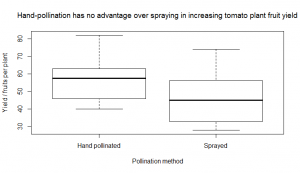1. Reaction rate plot
```# Simple version, no expression() malarkey

plot(
A405 ~ t,
data = reaction.rate,
xlab = "t / min",
ylab = "Absorbance (405 nm)",
main = "Accumulation of product in the assay is linear for 30 min"
)

# More complex version with subscript

plot(
A405 ~ t,
data = reaction.rate,
xlab = "t / min",
ylab = expression(A),
main = "Accumulation of product in the assay is linear for 30 min"
)```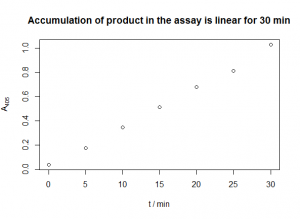1. Fly agaric plot.
```# Simple version, no expression() malarkey

plot(
Basidiocarps ~ Woodland,
data = fly.agarics,
xlab = "Woodland type",
ylab = "Basidiocarps / per hectare",
main = "Fly agaric basidiocarps are more common in birch woodland"
)

# More complex version, with italics and superscripts

plot(
Basidiocarps ~ Woodland,
data = fly.agarics,
xlab = "Woodland type",
ylab = expression("Basidiocarps"/ha^-1),
main = expression(italic("Amanita muscaria") * " basidiocarps are more
common in birch woodland")
)```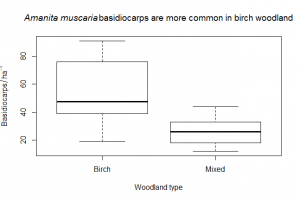1. Enzyme kinetic plot. The y-label is a bit tricky: ideally, you’d want to be able to write `expression(v/µmol*min^-1)` and perhaps this is what you tried. Unfortunately, the `*` in `expression()` just juxtaposes symbols, ignoring (and removing) any whitespace, even when it’s significant. Hence we have to add it manually inside a string `"µmol "`.
```# Simple version with default limits

plot(
v ~ S,
data = enzyme.kinetics,
xlab = "[NPP] / mM",
ylab = expression(v/"µmol " * min^-1),
main = "Acid phosphatase shows saturation kinetics on the substrate NPP",
ylim = c(0,10),
xlim = c(0,max(enzyme.kinetics\$S))
)

# Complex version, setting the limits nicely

plot(
v ~ S,
data = enzyme.kinetics,
xlab = "[NPP] / mM",
ylab = expression(v/"µmol " * min^-1),
main = "Acid phosphatase shows saturation kinetics on the substrate NPP",
ylim = c(0,10),
xlim = c(0,max(enzyme.kinetics\$S))
)```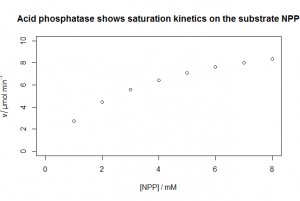1. Asellus gill movement plot.
```asellus.gills<-read.csv("H:/R/asellus_gills.csv")
plot(
Gill.movements ~ Water.quality,
data = asellus.gills,
xlab = "Water quality",
ylab = expression("Gill movements "/ min^-1),
main = expression("Water fleas (" * italic("Asellus") * "sp.) breathe harder in stagnant water")
)```

This site uses Akismet to reduce spam. Learn how your comment data is processed.